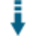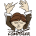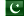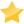# PHP Distance Calculation using Latitude and Longitude: Calculate the distance between two world locationsDownload .zip
 InfoExampleView files (3)Download .zip Reputation Support forum (2) Blog Links
 Ratings Unique User Downloads Download Rankings Not enough user ratings Total: 137 All time: 9,216 This week: 88Version License PHP version Categories distancecalculator 1.0 The PHP License 5 PHP 5, Geography
Description

Author

This class can calculate the distance between two world locations.

It can take the latitude and longitude coordinates of two locations in the worlds and calculates the distance between them.

The calculated distance can be returned as a string with the distance and optionally the distance unit in miles, kilometers, yards, meters and feets.Performance Level
Name: Classes: Tassadduq Hussain `` 1 package by Tassadduq HussainPakistan 36 4297 55 in Pakistan5307 in PakistanLevel 1## Example

 ``` lat1 = 33.6437323; \$dc->long1 = 72.9585014; //latitude and logtitude of point 2 \$dc->lat2 = 33.6655587; \$dc->long2 = 73.0022321; //define the unit of distance you want to get get data, default is 'miles' \$dc->type = 'km'; //choose if you want to display the selected unit type with the distance value, default is 'true' \$dc->show_type = false; //calculate and get the distance \$distance = \$dc->getDistance(); //show the calculated distance echo \$distance; ```

# DistanceCalculator

Calculate the distance between two points in Miles, Kilometers, Yards, Meters and Feets

Example

``````//includee the class
require_once('DistanceCalculator.Class.php');

//initiate class
\$dc = new DistanceCalculator();

//latitude and logtitude of point 1
\$dc->lat1 = 33.6437323;
\$dc->long1 = 72.9585014;

//latitude and logtitude of point 2
\$dc->lat2 = 33.6655587;
\$dc->long2 = 73.0022321;

//define the unit of distance you want to get get data, default is 'miles'
\$dc->type = 'km';

//choose if you want to display the selected unit type with the distance value, default is 'true'
\$dc->show_type = false;

//calculate and get the distance
\$distance = \$dc->getDistance();

//show the calculated distance
echo \$distance;
``````Files
File Role DescriptionDistanceCalculator.Class.php Class Class sourceexample.php Example Example scriptREADME.md Doc. Documentation

 All time: 9,216 This week: 88About usAdvertise on this siteSite mapNewsletterStatisticsSite tipsPrivacy policyContact
For more information send a message to `info at phpclasses dot org`.